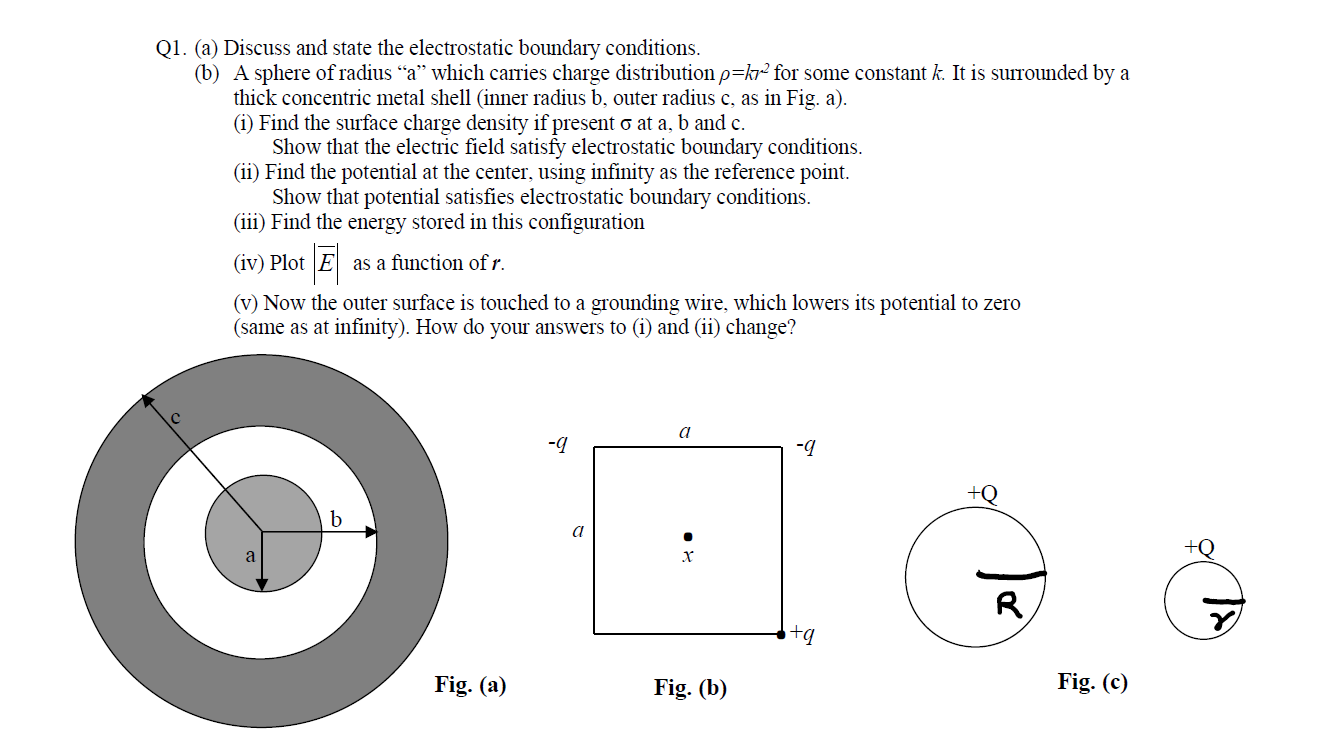### Create an Account

Home / Questions / Discuss and state the electrostatic boundary conditions. (b) A sphere of radius “a” which...

# Discuss and state the electrostatic boundary conditions. (b) A sphere of radius “a” which carries charge distribution p=kr for some constant k. It is surrounded by a thick concentric metal shell

Discuss and state the electrostatic boundary conditions. (b) A sphere of radius “a” which carries charge distribution p=kr for some constant k. It is surrounded by a thick concentric metal shell (inner radius b, outer radius c, as in Fig. a). (1) Find the surface charge density if present o at a, b and c. Show that the electric field satisfy electrostatic boundary conditions. (ii) Find the potential at the center, using infinity as the reference point. Show that potential satisfies electrostatic boundary conditions. (iii) Find the energy stored in this configuration (iv) Plot E as a function of r. (v) Now the outer surface is touched to a grounding wire, which lowers its potential to zero (same as at infinity). How do your answers to (i) and (ii) change?May 31 2021 View more View LessSubscribe To Get Solution